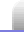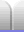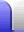# Pythagoras and Trigonometry Revision Questions

### 1.

A. A ship sails 20 km due north and then 35 km due east. How far is it from its starting point?

Use Pythagoras' Theorem:

a2 + b2 = c2

202 + 352 = c2

400 + 1225 = 1625 = c2

√ 1625 = c = 40.31 km

Note that the question simply asks how far it is from its starting point, so no bearing is required, just the distance. Don't forget the units! (In this case "km".)

B. At what bearing will the ship need to travel to get back to its starting point (to the nearest degree)?

The ship has traveled north and east, so it will have to go south and west to get back, so we know the bearing will be somewhere in the south west quadrant, from 180° to 270°. (In other words, the answer is a reflex angle.) We use trignometry to find the interior angle of the triangle, then subtract that from 270°:

tan Ø = opposite / adjacent

tan Ø = distance travelled north / distance travelled east

tan Ø = 20 / 35

Ø = tan-1( 20 / 35 )

Ø = 30° (0dp)

bearing = 270° - 30° = 240°

Remember, if our answer is outside the southwest quadrant we've made a mistake somewhere. Don't forget the degrees symbol.

### 2.

An 18 cm long piece of wire is bent into a U shape by bending it through 90° 3 cm from one end, and another 90° 3 cm further along the wire. What is the distance between the ends?

The area enclosed by the wire can be thought of as a square with an adjoining right-angle triangle, with the line between the ends of the wire the hypotenuse of the triangle. Use Pythagoras' theorem, taking off the length of the three sides of the square to find the length of the triangle:

a2 + b2 = c2

32 + (18 - 3 - 3 - 3)2 = c2

32 + 92 = c2

9 + 81 = 90 = c2

√ 90 = c = 9.49 cm

### 3.

A girl is flying a kite with a string length of 60 metres. Ignoring the girl's height, if the string is taut and makes an angle of 71° to horizontal, how high is the kite?

Use trigonometry:

sin ø = opposite / hypotenuse

sin ø = height of kite / string length

sin (71°) = height of kite / 60

60 * sin (71°) = height of kite

height of kite = 56.73 m

### 4.

When a particular 2 m ladder is leaned against a wall, its instructions say it must be placed at a 4:1 angle ratio or steeper to prevent its feet from slipping outward (ie, for every cm the base is out from the wall, the top must be at least 4 cm up the wall). What is the minimum height the ladder will reach up a wall without risking slipping?

Use Pythagoras' theorem:

a2 + b2 = c2

Remember that we square the 4x, not square the x then multiply by 4.

x2 + (4x)2 = 22

x2 + 16x2 = 17x2 = 4

x2 = 4 / 17

x = √ ( 4 / 17 ) = 0.485

height up wall = 4x = 1.94 m

### 5.

An archer defending a castle knows he has an accurate/effective range of 80 m for a human-sized target and 160 m for an elephant-sized target. (FWIW Wikipedia says An archer could hit a person at 165 m (180 yards) "part of the time" and could always hit an army.) He also knows that when he is standing on the castle wall, a marker stone 10 m out from the castle wall makes an angle of about 64° below horizontal, and a marker stone 50 m out makes an angle of about 22° below horizontal.

A. How high is the castle wall?

Use trigonometry for each of the marker stones then find an average of the two values:

tan ø = opposite / adjacent

tan ø = height of castle wall / distance of marker stone

tan (64°) = height of castle wall / 10

10 * tan (64°) = height of castle wall = 20.5 m (estimate 1)

tan (22°) = height of castle wall / 50

50 * tan (22°) = height of castle wall = 20.2 m (estimate 2)

Average = ( 20.2 + 20.5 ) / 2 = 20.35 m

B. With your answer in whole degrees, what angle below horizontal will elephants be when the archer can start firing at them? (Consider: To get an answer in whole degrees should the number be rounded up, down, or to the nearest whole number?)

Use trigonometry:

tan ø = opposite / adjacent

tan ø = height of castle wall / distance of elephant

tan ø = 20.35 / 160

ø = tan-1 ( 20.35 / 160 ) = 7.25°

We should arguably round up to ensure the target is actually within range - if we rounded down the target would be just outside the maximum range.

Ø =

C. With your answer in whole degrees, what angle below horizontal will human targets be when they come within range?

Use trigonometry:

tan ø = opposite / adjacent

tan ø = height of castle wall / distance of human

tan ø = 20.35 / 80

ø = tan-1 ( 20.35 / 80 ) = 14.27°

Again, we should arguably round up to ensure the target is within range.

Ø = 14°

If our answers are more than 22° we've made a mistake, since that's the angle that objects at 50 m will be at.Home Astronomy Chemistry Electronics & Computers Mathematics Physics Field Trips Turn on javascript for email link